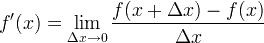# RD Sharma Solutions Class 11 Derivatives

## RD Sharma Solutions Class 11 Chapter 30

Derivatives are a fundamental tool of calculus. It is mainly applied when there is the certain varying quantity and the rate of change is not constant. It is mainly used in measuring the sensitivity of one variable with respect to another variable.

For the function f, its derivative is said to be f'(x). The derivative formula is given as,For example- Find the fourth derivative of f (x) = 2x5

Now by applying the formulaef (4) (x) = [2x5]”” = [10x4]”’ = [40x3]” = [120x2]’ = 240x

Practice problems on derivatives through solved RD Sharma solutions to have a better understanding of the topic.

 Chapter 30  Derivatives Derivatives Exercise 30.1 Derivatives Exercise 30.2 Derivatives Exercise 30.3 Derivatives Exercise 30.4 Derivatives Exercise 30.5